# Continued fraction

(diff) ← Older revision | Latest revision (diff) | Newer revision → (diff)

An expression of the form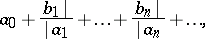(1)

where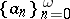(2)

and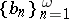(3)

are finite or infinite sequences of complex numbers. Instead of the expression (1) one also uses the notationThe continued fraction of the sequence (2) is defined as the expressionFor every continued fraction (1) the recurrence equations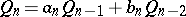with the initial conditionsdetermine two sequences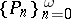andof complex numbers. As a rule, it is assumed that the sequences (2) and (3) are such that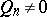for all,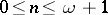. The fractionis called the-th convergent of the continued fraction (1). Here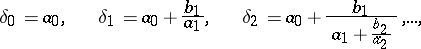moreover,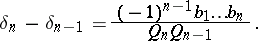It is convenient to denote the-th convergent of the continued fraction of the sequence (2) byThese convergents satisfy the following equalities: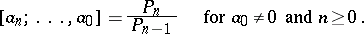If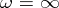and the sequence of convergents of (1) converges to some limit, then the continued fraction (1) is called convergent and the numberis its value. If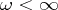, that is, the continued fraction is finite, then its value is defined as the last of its convergents.

If all terms of the sequences (2) and (3), except possibly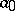, are positive real numbers, and ifis real, then the sequenceof convergents of even order of (1) increases, and the sequence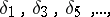of convergents of odd order decreases. Here a convergent of even order is less than the corresponding convergent of odd order (see ).

Ifis the sequence of complex numbers for whichthen the expression (1) is called an expansion of the number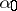in a continued fraction. Not every continued fraction converges, and the value of a continued fraction is not always equal to the number from which it is expanded. There are a number of criteria for the convergence of continued fractions (see, for example,  and ):

1) Suppose that, that all terms of the sequences (2) and (3) are real numbers, and that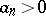for all natural numbersfrom some term onwards. If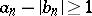for such, then the continued fraction (1) converges.

2) Suppose thatand that all terms of the sequence (2) beginning with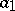are positive. Then the continued fraction of the sequence (2) converges if and only if the seriesdiverges (Seidel's theorem).

The continued fraction of a sequence (2) is called regular if all its terms (except possibly) are natural numbers,is an integer andfor. For every real numberthere exists a unique regular continued fraction with value. This fraction is finite if and only ifis rational (see , , ). An algorithm for the expansion of a real numberin a regular continued fraction is defined by the following relations(4)

where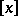denotes the integral part of.

The numbersand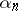defined by (4) are called, respectively, the complete and incomplete quotients of orderof the expansion ofin a continued fraction.

Around 1768 J. Lambert found the expansion ofin a continued fraction: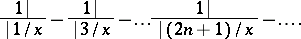Under the assumption that this continued fraction converges, A. Legendre proved that its value for rational values ofis irrational. It should be mentioned that in this way he proved the irrationality of the number(see ).

L. Euler found in 1737 that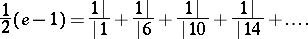A real numberis an irrational root of a polynomial of degree 2 with integer coefficients if and only of the incomplete quotients of the expansion ofin a continued fraction from some term onwards are repeated periodically (the Euler–Lagrange theorem, see  and ). At present (1984) expansions in regular continued fractions of algebraic numbers of degree 3 and higher are not known. The assertion that the incomplete quotients of the expansion ofin a continued fraction are bounded has not been proved.

Regular continued fractions are a very convenient tool for the approximation of real numbers by rational numbers. The following propositions hold:

1) Ifandare neighbouring convergents of the expansion of a numberin a regular continued fraction, then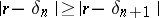andwhere in the latter case equality holds only when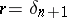.

2) For two neighbouring convergents of the expansion of a numberin a regular continued fraction, at least one of them satisfies the inequality:3) Ifandare integers,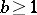, ifis a real number, and ifthen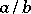is a convergent of the expansion ofin a regular continued fraction.

4) If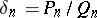is a convergent of the expansion of a numberinto a regular continued fraction, then for any integersandit follows from,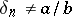and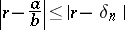that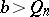(theorem on the best approximation).

The first twenty-five incomplete quotients of the expansion of the numberin a regular continued fraction are: 3, 7, 15, 1, 292, 1, 1, 1, 2, 1, 3, 1, 14, 2, 1, 1, 2, 2, 2, 2, 1, 84, 2, 1, 1.

The first five convergents of the expansion ofin a regular continued fraction are: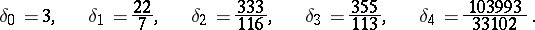Therefore,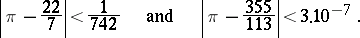There exist several generalizations of continued fractions (see, e.g., ).